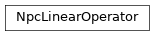# NpcLinearOperator¶

Inheritance DiagramMethods

 `NpcLinearOperator.__init__`() Return the hermitian conjugate of self Calculate the action of the operator on a vector vec. Contract self to a matrix.

Class Attributes and Properties

class tenpy.linalg.sparse.NpcLinearOperator[source]

Bases: `object`

Prototype for a Linear Operator acting on `Array`.

Note that an `Array` implements a matvec function. Thus you can use any (square) npc Array as an NpcLinearOperator.

dtype

The data type of its action.

Type

np.type

acts_on

Labels of the state on which the operator can act. NB: Class attribute.

Type

list of str

matvec(vec)[source]

Calculate the action of the operator on a vector vec.

Note that we don’t require vec to be one-dimensional. However, for square operators we require that the result of matvec has the same legs (in the same order) as vec such that they can be added. Note that this excludes a non-trivial qtotal for square operators.

to_matrix()[source]

Contract self to a matrix.

If self represents an operator with very small shape, e.g. because the MPS bond dimension is very small, an algorithm might choose to contract self to a single tensor.

Returns

matrix – Contraction of the represented operator.

Return type

`Array`